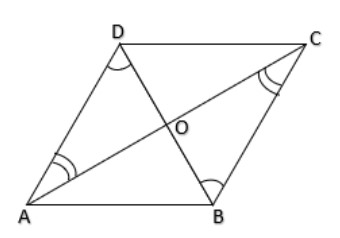QUESTION

# A question is asked to name the quadrilaterals whose diagonals bisect each other.Answer so given is Parallelogram; rhombus; square; rectangle.Choose whether the answer to the question is True or False.(i) True(ii) False

Hint- The main hint for this question is that we have to just check for the parallelogram property to others because all the others are just subset for the parallelogram, so if parallelogram satisfies the property that diagonals bisect each other, then all the others namely rhombus, square and rectangle will also satisfy that.

Let us suppose a parallelogram ABCD with diagonals AC & BD and O is the point of intersection of AC & BD.If diagonals AC & BD bisect each other at O then OA = OC & OB = OD
In parallelogram ABCD, AD || BC with transversal BD so $\angle ODA = \angle OBC$ -(1) as they are Alternate interior angles.
Also, AD || BC with transversal AC so $\angle OAD = \angle OCB$ -(2) as they are Alternate interior angles.

Now in $\Delta AOD$ and $\Delta BOC$
$\angle OAD = \angle OCB$ (From (2))
AD = CB (Opposite sides of a Parallelogram are equal)
$\angle ODA = \angle OBC$ (From (1))
$\Delta AOD \cong \Delta BOC$ (Angle Side Angle Rule of Congruence)
So, OA = OC & OB = OD (Congruent Parts of Congruent Triangles)
Hence, we can say that the diagonals of a parallelogram bisect each other.
Now, we know that rhombus, square and a rectangle are all the types of parallelogram so if in a parallelogram the diagonals bisect each other, then the diagonals of rectangle, square and rhombus also bisect each other.
Hence the answer to this question is True that the diagonals of Parallelogram, rhombus, square and rectangle bisect each other.
$\therefore$ Option A. True is our correct answer.

Note- Just keep in mind for such questions either check one and check whether all satisfy the same property or check for all one by one and then compare. Also remember the basic property that Diagonals of parallelograms bisect each other. Diagonals of Rectangle also bisect each other. If they bisect each other at right angles, then the figure can be a square or a rhombus. If the diagonals are of equal length, then the figure is a square and if not then it’s a rhombus. In the conclusion, diagonals of parallelogram, Rhombus, square and rectangle bisect each other.